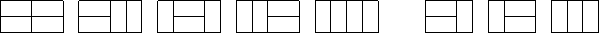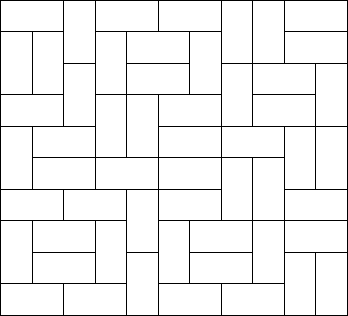## poj 2411 Mondriaan’s Dream

2019年11月08日 算法 ⁄ 共 1846字 ⁄ 字号 评论关闭

dp[i][j]:有多少种方法，可以使得第i行的状态为j

dp[i][j]=sum{dp[i-1][k],k可以通过合法变化变成状态j}

0:该位置空余

1:该位置被占

```#include<iostream>
#include<cstring>
using namespace std;
long long dp[1<<11];
int n,m;
void dfs(int s,int row,int line,long long val)
{
int i,k;
k=m-1;
dp[row][line]+=val;
for(i=s;i<k;i++)
if((line>>i&1)==0)
if((line>>i+1&1)==0)
dfs(i+2,row,line|(1<<i)|(1<<i+1),val);
}
int main()
{
int i,j,k;
while(cin>>n>>m)
{
if(n==0&&m==0)
break;
memset(dp,0,sizeof(dp));
dfs(0,0,0,1);
k=1<<m;
for(i=1;i<n;i++)
for(j=0;j<k;j++)
dfs(0,i,k-1^j,dp[i-1][j]);
cout<<dp[n-1][k-1]<<endl;
}
return 0;
}```

POJ - 2411

 Time Limit:3000MS Memory Limit:65536KB 64bit IO Format:%I64d & %I64u

Description

Squares and rectangles fascinated the famous Dutch painter Piet Mondriaan. One night, after producing the drawings in his 'toilet series' (where he had to use his toilet paper to draw on, for all of his paper was filled with squares
and rectangles), he dreamt of filling a large rectangle with small rectangles of width 2 and height 1 in varying ways.Expert as he was in this material, he saw at a glance that he'll need a computer to calculate the number of ways to fill the large rectangle whose dimensions were integer values, as well. Help him, so that his dream won't turn into a nightmare!

Input

The input contains several test cases. Each test case is made up of two integer numbers: the height h and the width w of the large rectangle. Input is terminated by h=w=0. Otherwise, 1<=h,w<=11.

OutputFor each test case, output the number of different ways the given rectangle can be filled with small rectangles of size 2 times 1. Assume the given large rectangle
is oriented, i.e. count symmetrical tilings multiple times.

Sample Input

```1 2
1 3
1 4
2 2
2 3
2 4
2 11
4 11
0 0
```

Sample Output

```1
0
1
2
3
5
144
51205
```

Source